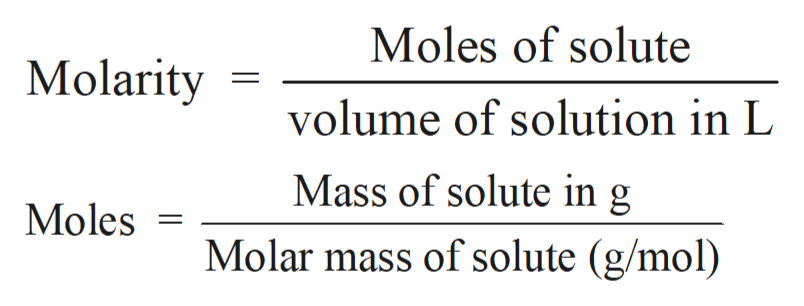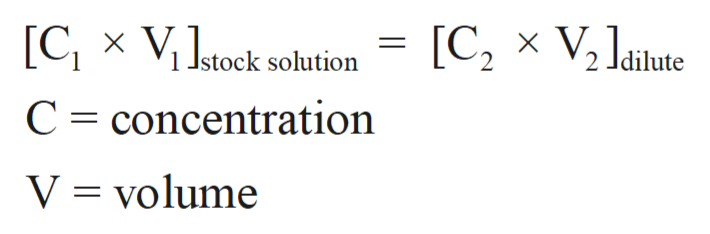if you are making a set of standards from a 10.0mg/L standard, show the calculation and explain how you acutally make up 2mL of 4.00mg/mL standard.

Question

if you are making a set of standards from a 10.0mg/L standard, show the calculation and explain how you acutally make up 2mL of 4.00mg/mL standard.

Step 1

Solution stoichiometry involves the calculation of concentration of solutions in the given conditions of volumes, moles etc.

Step 2

Solution stoichiometry is mainly based on the calculation of moles and volumes. These two values are used to calculate the molarity of solution. The relation between moles, volume and molarity is as given below;help_outlineImage TranscriptioncloseMoles of solute Molarity volume of solution in L Mass of solute in g Moles Molar mass of solute (g/mol) fullscreen
Step 3

For the concentration of dilute solution:

Concentration of stock solution = 10 mg/mL

Volume of stock solu...help_outlineImage Transcriptionclose[CX ViJstock solution [C2 V2 Jaiute C concentration V volume fullscreen

Want to see the full answer?

See Solution

Want to see this answer and more?

Our solutions are written by experts, many with advanced degrees, and available 24/7

See Solution
Tagged in

Solutions